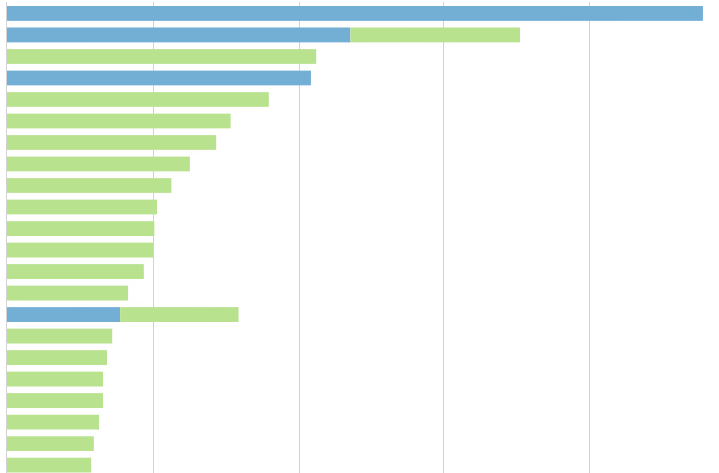Stacked Bar Chart Sorting Incorrectly

I have a stacked bar chart and would like to sort it in decending order based on the sum of the metric used in the chart.  Instead of sorting by the total for the y axis category, it's sorting based on the total for category/series combination.  The screenshot below shows an example where the sorting only factors the y axis category/series with the greatest value instead of the y axis category total across all of the series'